0反面
1正面

## 3. 整数与补码表示法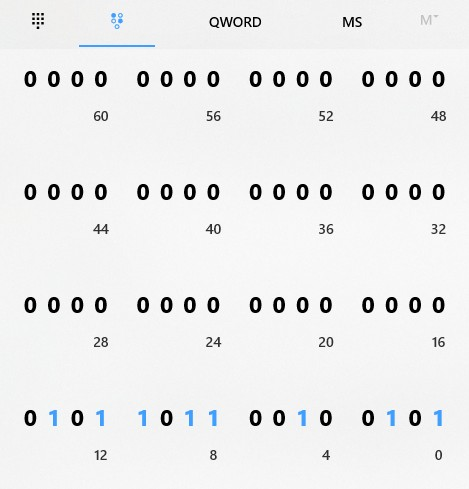1. 对于正数和 0 来说，它的补码即这个数字原始的二进制表达。
2. 对于负数，它的补码是一个能与它的绝对值“互补”的数。

### 3.1 钟表的运算模型

“补码”本质上是依赖某种类似钟表的模型设计的规则，假设我们有一个只有秒针的表，如图。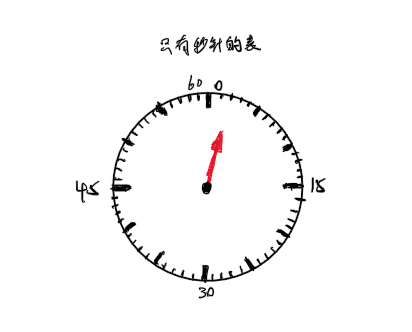(“在第几个刻度出发” + “变化的刻度数”) 除以 “表盘总共的刻度数” 得到的 余数

$$(0 + 20 + 30 + 20) \space mod \space 60 = 10$$

### 3.2 刻度的分配策略

$$(1+59)\ mod\ 60 = 0 \\ (2+58)\ mod\ 60 = 0 \\ (3+57)\ mod\ 60 = 0 \\ ... \\ (29+31)\ mod\ 60 = 0 \\ (30+30)\ mod\ 60 = 0$$

$$(1+(-1)) = 0 \\ (2+(-2)) = 0 \\ (3+(-3)) = 0 \\ ... \\ (29+(-29)) = 0 \\ (30+(-30)) = 0$$

1. 钟表的零刻度分给整数中的 0
2. 刻度 1 ~ 29 分给整数中的 1 ~ 29
3. 刻度 59 ~ 30 分给整数中的 -1 ~ -30### 3.3 实际的补码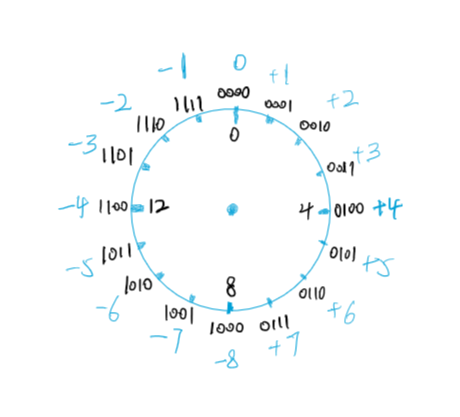01117
01106
......
00102
00011
00000
1111−1
1110−2
......
1001−7
1000−8

### 3.4 计算器的 “程序员” 模式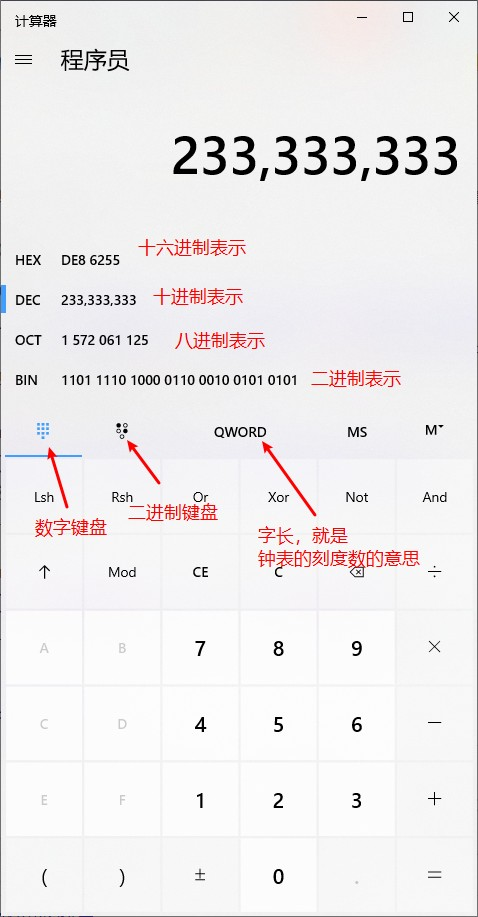Byte（字节）-82^8
Word（字）-162^16
DWord（双字）Double Word322^32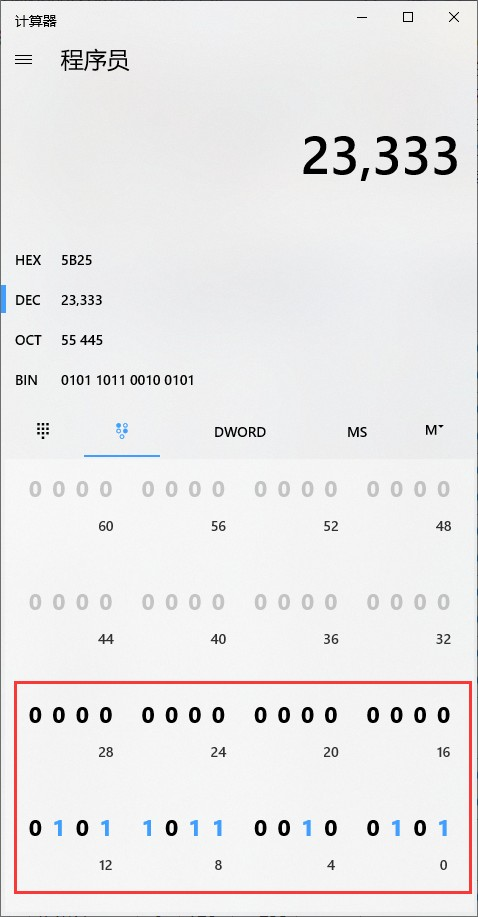## 4. 小数与浮点表示法

### 4.1 “乘2”与移位

$$101_2 = 1*2^2+0*2^1+1*2^0 = 5 \\ 1010_2 = 1*2^3+0*2^2+1*2^1+0*2^0\\ = 10_{10} = 5*2$$

### 4.2 浮点数基本结构

32 个二进制位的分配如下：

1. 分一个给符号位，符号位为 1 时代表负、为 0 时代表正。
2. 然后分 8 个位给指数，这个数参与最后的求值过程。
3. 剩下还有 23 个位，分给有效数位，有效数位本身有 24 位，规则规定第一位一定是1，那就不需要再储存，省下1位，剩下23位实际储存。

$$value = sign\times fraction \times 2^{exponent}$$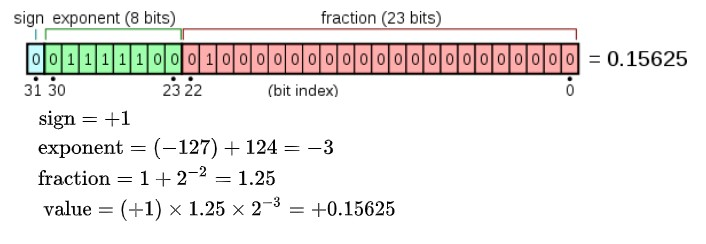### 4.3 浮点数类型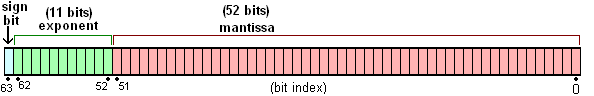### 4.4 精度问题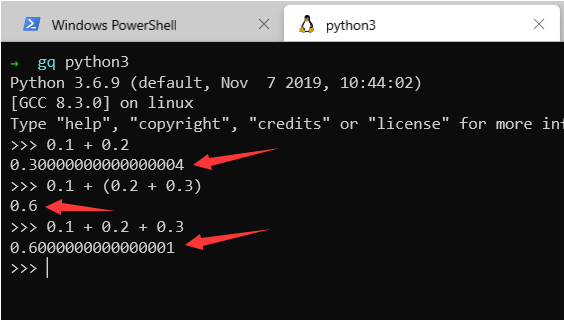Python 的数字默认用了双精度浮点数，也就是说，转换成二进制后的精确值只能保留到小数点 52 位，0.1 与 0.2 对应的二进制的值如下。

0.1 -> 0.0001100110011001100110011001100110011001100110011001
0.2 -> 0.0011001100110011001100110011001100110011001100110011

## 5. 参考资料

1.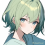idealclover

我记得有种说法说补码可以用同余来理解的~

不过毕竟学了好长时间了忘差不多了XD

1.qing

对对，我这里的钟表其实就是同余，参考链接的可汗学院的那篇文章我觉得说得超级形象~
这么一说想起来之前看的一个知乎，链接再补上